# Статьи журнала - Вестник Южно-Уральского государственного университета. Серия: Математика. Механика. Физика

Все статьи: 663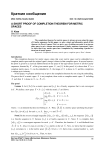A short proof of completion theorem for metric spaces

Kaya U.

Краткое сообщение

The completion theorem for metric spaces is always proven using the space of Cauchy sequences. In this paper, we give a short and alternative proof of this theorem via Zorn’s lemma. First, we give a way of adding one point to an incomplete space to get a chosen non-convergent Cauchy sequence convergent. Later, we show that every metric space has a completion by constructing a partial ordered set of metric spaces.

Бесплатно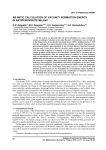Ab initio calculation of vacancy formation energy in antiperovskite Mn3GaC

Baigutlin D.R., Zagrebin M.A., Sokolovskiy V.V., Buchelnikov V.D.

Статья научная

In this article, we presented the ab initio calculation of vacancy formation energy according to Schottky in the alloy Mn3GaC. Calculations were carried out in the frameworks of the density functional theory (DFT), implemented in VASP software package. For approximation of the exchange-correlation functional, the generalized gradient approximation in the Perdew-Burke-Ernzerhof formulation was used. It was shown that for the alloy under research, the most energetically favorable formation of a vacancy is in the place of C atom; formation of vacancies in places of Mn atoms is also beneficial, whereas the Ga vacancies are energetically unfavorable. Also, the concentration of vacancies at a finite temperature was calculated. It was shown that Mn and C vacancies have almost identical equilibrium concentration at a nonzero temperature; at that, the concentration of Ga vacancies is negligibly small. In addition, elastic moduli for various magnetic orderings (ferromagnetic, noncollinear, and antiferromagnetic) in the alloy under research were calculated. Using the quasi-harmonic Debye model, the Helmholtz free energy curves were constructed. Using these curves, it was also shown that Schottky monovacancies do not destabilize the ferromagnetic phase. Stability of the ferromagnetic phase is due to the large contribution of magnetic entropy to the Helmholtz free energy for the alloy under research.

Бесплатно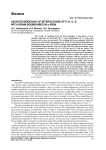Ab initio modeling of interactions of P, H, C, S with grain boundaries in a-iron

Verkhovykh A.V., Mirzoev A.A., Dyuryagina N.S.

Статья научная

The results of modeling from the first principles of interaction of non-metallic impurities of interstitial (H, C) and substitutional (P, S) with grain boundaries in α-iron are presented. The modeling has been conducted within the framework of the density functional theory (DFT) by the full-potential linearized augmented plane waves (FP LAPW) method with consideration to the generalized gradient approximation (GGA'96) in the WIEN2k software package. Three grain boundaries of the slope Σ3 (111), Σ5 (210) and Σ5 (310) are studied. The supercells of the tilt grain boundaries using the coincidence site lattice model is constructed. The values of the energy characteristics of various grain boundaries with impurities are influenced by a number of factors, namely, the volume of the Voronoi polyhedron per impurity, magnetic moments, and the symmetry of the surrounding matrix. The results show that symmetric grain boundaries Σ3 (111) and Σ5 (310) are embrittled by phosphorus, hydrogen, and sulfur, while carbon strengthens interatomic bonds at the grain boundary, which coincides with the data available in the work. In the case of an asymmetric grain boundary Σ5 (210), phosphorus and hydrogen weaken bonds at the grain boundary, while sulfur strengthens them. This is primarily explained by the geometry of the surrounding matrix. The magnetic moments at the impurity atoms are very small and, in most cases, are antiparallel to the magnetic moments at the neighboring Fe atoms.

Бесплатно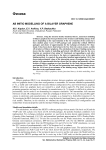Ab initio modelling of a bilayer graphene

Kaplun M.V., Anikina E.V., Beskachko V.P.

Статья научная

Using the electron density functional theory, numerical modelling of bilayer graphene has been performed. The structure and binding energy of the layers depending on the representation of the wave function of the system have been studied: plane waves (VASP package) and atomic-like orbitals (SIESTA package); and choice of approximation for the exchange-correlation (XC) functional. It has been shown that being in the free form the system creates a stable AB structure of bilayer graphene. The calculation of the layer binding energy has shown that the results of modelling performed with different basis for the wave function are consistent when using XC functionals corresponding to each other and considering the correction to the basis set superposition error in the tight binding method. As expected, the generalized gradient approximation (GGA) has shown underestimated values of the interaction energy of graphene layers. Comparison with experimental data has shown that the energy and geometric characteristics of bilayer graphene are best described by the local electron density approximation (LDA). The 2nd and 3rd generation semi-empirical Grimme corrections for GGA have given estimates of the binding energy higher than LDA, but also close to the experimental results.

Бесплатно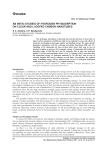Ab initio studies of hydrogen physisorption on clear and Li-doped carbon nanotubes

Anikina E.V., Beskachko V.P.

Статья научная

The hydrogen adsorption on internal and external surfaces of clear and Li-doped carbon nanotubes of different radii are investigated to assess the effects of concavity and doping on hydrogen uptake and binding energy. We make density functional calculations with the exchange-correlation functionals PBE and CA. Modeling of H2 adsorption on clear carbon tubes shows that only in case of internal sorption on narrow (5,5) nanotube energy of adsorption fall within the desirable range of 300-400 meV per H2 molecule. But in this case hydrogen uptake is too low and constitutes about 1,6 wt %. Doping with Li atom increases the adsorption energy of hydrogen molecule by 30-100 meV and in case of external sorption this energy enlarges several times. Nevertheless, the optimal range of binding energy can be achieved only in case of hydrogen adsorption inside quite narrow (5,5) and (7,7) Li-doped nanotubes.

Бесплатно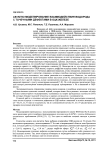Ab initio моделирование взаимодействия водорода с точечными дефектами в ОЦК-железе

Урсаева Анастасия Владимировна, Ракитин Максим Сергеевич, Рузанова Галина Евгеньевна, Мирзоев Александр Аминулаевич

Статья научная

Проведено ab initio моделирование взаимодействия водорода с точечными дефектами в ОЦК-железе. Определено равновесное положение и энергия захвата водорода в ячейке с вакансией. Показано, что связь водорода с комплексом дефектов вакансия + атом замещения в основном определяется взаимодействием водорода с вакансией.

Бесплатно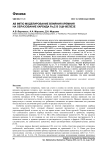Ab initio моделирование влияния кремния на образование карбида Fe3C в ОЦК-железе

Верховых А.В., Мирзоев А.А., Мирзаев Д.А.

Статья научная

Представлены результаты первопринципного моделирования влияния кремния на энергию формирования цементита и парциальную энтальпию. Моделирование проводилось в рамках теории функционала плотности (DFT) полнопотенциальным методом линеаризованных присоединенных плоских волн (FP LAPW) с учетом обобщенного градиентного приближения (GGA’96) в программном пакете WIEN2k. Были изучены различные концентрации примеси кремния в цементите, а именно, 1,6, 3,2 и 6 ат. % как в позиции замещения атома железа (S и G-позиции), так и атома углерода (С-позиция). Была выполнена объемная оптимизация структур. Найдены равновесные параметры решетки как цементита без примеси, так и в присутствии кремния, которые отлично согласуются с экспериментальными и теоретическими данными. Энергия формирования для концентрации 3,2 ат. % в С-позиции оказалась -0,03 эВ, что может говорить о стабилизации цементита. Но при этом парциальная энтальпия для всех положений кремния положительна, и значит, кремний остается в твердом растворе ОЦК-Fe, что находится в хорошем согласии с результатами других теоретических и экспериментальных работ. Было получено, что чем больше концентрация кремния, тем ниже средний магнитный момент на атомах железа.

БесплатноAb initio моделирование энергии формирования границы зерна в ОЦК-железе

Верховых Анастасия Владимировна, Мирзоев Александр Аминулаевич

Статья научная

С помощью программного пакета WIEN2k проведено первопринципное моделирование границы зерна Σ5 ОЦК-железа. Получены оптимальные параметры и рассчитаны энергии формирования границы зерна для двух углов разориентировки.

Бесплатно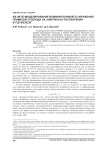Ab-initio моделирование влияния ближнего окружения примесей углерода на энергию их растворения в ГЦК-железе

Ридный Ярослав Максимович, Мирзоев Александр Аминулаевич, Мирзаев Джалал Аминулович

Статья научная

В программном пакете WIEN2k проведено первопринципное моделирование равновесной структуры и свойств ГЦК-железа. Получены оптимальные параметры, позволяющие построить наиболее точную модель. Произведено вычисление энергий растворения атомов углерода, их взаимного расположения и вклада упругих эффектов в энергию системы для немагнитного (НМ) и антиферромагнитного двухслойного (АФМД) состояний ГЦК железа.

Бесплатно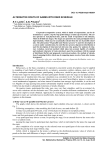Alternative routs of games with rigid schedule

Larkin E.V., Privalov A.N.

Статья научная

Corporative-competitive system, which is inside of corporations, can be determined as a “game”, step-by-step performing a certain type of activity. The system operates in real physical time, and the result of operation is the distance, which is divided into stages. The stages are passed by the team participants due to rigid schedule, which may be occasionally selected from the set of possible schedules. The abstraction “M-parallel semi-Markov process” is used for description of a system under consideration. In semi-Markov process degenerate distribution is used for description of time intervals between relay points. For analysis of relay-race evolution, recurrent method which takes into account rigidity of schedule and stochastic character of route selection is used. In accordance with the concept of distributed forfeit and proposed recurrent procedure, the method of calculation of summing forfeit, which one of competing teams receives from other teams, is proposed.

Бесплатно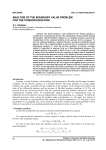Analysis of the boundary value problem for the Poisson equation

Ushakov A.L.

Статья научная

The mixed boundary value problem for the Poisson equation is considered in a bounded flat domain. The continuation of this problem through the boundary with the Dirichlet condition to a rectangular domain is carried out. Consideration of the continued problem in the operator form is proposed. To solve the continued problem, a method of iterative extensions is formulated in an operator form. The extended problem in operator form is considered on a finite-dimensional subspace. To solve the previous problem, an iterative extension method is formulated in operator form on a finite-dimensional subspace. The continued problem is presented in matrix form. To solve the continued problem in matrix form, the method of iterative extensions in matrix form is formulated. It is shown that in the proposed versions of the method of iterative extensions, the relative errors converge in a rate that is stronger than the energy norm of the extended problem with the rate of geometric progression. The iterative parameters in these methods are selected using the minimum residual method. Conditions are indicated that are sufficient for the convergence of the applied iterative processes. An algorithm is written that implements the method of iterative extensions in matrix form. In this algorithm, the iterative parameters are automatically selected and the stopping criterion is indicated when the estimate of the required accuracy is reached. Examples of application of the method of iterative extensions for solving problems on a computer are given.

Бесплатно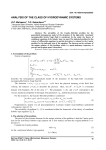Analysis of the class of hydrodynamic systems

Matveeva O.P., Sukacheva T.G.

Статья научная

The solvability of the Cauchy-Dirichlet problem for the generalized homogeneous model of the dynamics of the high-order viscoelastic incompressible Kelvin-Voigt fluid is considered. In the study, the theory of semilinear equations of the Sobolev type was used. The indicated problem for the system of differential equations in partial derivatives is reduced to the Cauchy problem for the indicated type of the equation. The theorem on the existence of the unique solution of this problem, which is a quasi-stationary trajectory, is proved, and its phase space is described.

Бесплатно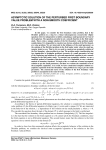Asymptotic solution of the perturbed first boundary value problem with a non-smooth coefficient

Tursunov D.A., Orozov M.O.

Статья научная

In this paper, we consider the first boundary value problem, that is the Dirichlet problem in a ring for a linear inhomogeneous second-order elliptic equation with two independent variables containing a small parameter in front of the Laplacian. The equation potential is not a smooth function in the field under study. There exists a unique solution of the first boundary value problem under consideration. It is impossible to construct an obvious solution of the first boundary value problem. We are interested in the influence of the small parameter on the solution of the Dirichlet problem in the field under study when the small parameter tends to zero. That is why we need to construct an asymptotic solution of the first boundary value problem in a ring. The problem under consideration has two singularities (a bisingular problem): presence of a small parameter in front of the Laplacian, and solution of a relevant unperturbed equation is not a smooth function in the field under study. To construct an asymptotic solution, we use a modified method of boundary functions since it is impossible to use a classical method of boundary functions. To begin with, we construct a formal asymptotic solution as per the small parameter, and then we evaluate the remainder term of the asymptotic expansion. As a result, we have constructed complete uniform asymptotic expansion of the first boundary value problem in a ring as per the small parameter. The constructed series of the solution of the first boundary value problem is asymptotic in the sense of Erdey.

Бесплатно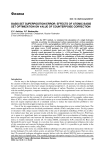Basis set superposition error: effects of atomic basis set optimization on value of counterpoise correction

Anikina E.V., Beskachko V.P.

Статья научная

Using the DFT method, we simulated the adsorption of a single hydrogen molecule on pristine low-dimensional carbon nanomaterials: carbon nanotubes (CNT), en-yne (CEY), and graphdiyne (GDY). For wave function decomposition, we employed two approaches: localized pseudoatomic orbitals (SIESTA package) and plane waves (VASP package). For CNT, CEY, GDY, and bulk carbon (graphite), we optimized atomic basis sets. Delta test of used DFT packages showed a good agreement for carbon: ∆С = 0,36 meV/atom. We demonstrated that after atomic basis set optimization the value of counterpoise (CP) correction of basis set superposition error (BSSE) in calculations of hydrogen adsorption energies reduces. Moreover, this CP correction could be by several times bigger than the corrected hydrogen adsorption energy. Therefore, to obtain reasonable results in weakly interacting systems, CP-corrected adsorption energies in the optimized PAOs are needed. In considered systems, hydrogen adsorption energies, which were calculated in this way, agree with the energies obtained using the BSSE-free plane-wave basis set.

Бесплатно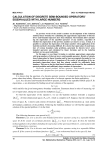Calculation of discrete semi-bounded operators’ eigenvalues with large numbers

Kadchenko S.I., Zakirova G.A., Ryazanova L.S., Torshina O.A.

Статья научная

In previous works of the article’s authors on development of the Galerkin method, linear formulas for calculating the approximate eigenvalues of discrete lower semi-bounded operators have been obtained. The formulas allow calculating the eigenvalues of the specified operators of any number, regardless of whether the eigenvalues of the previous numbers are known or not. At that, it is possible to calculate the eigenvalues with large numbers when application of the Galerkin method is becoming difficult. It is shown that eigenvalues of small numbers of various boundary-value problems, generated by discrete lower semi-bounded operators and calculated by linear formulas and by the Galerkin method, are in a good conformity. In this paper we use linear formulas to calculate approximate eigenvalues with large numbers of discrete lower semi-bounded operators. Results of calculation of eigenvalues by linear formulas and by known asymptotic formulas for two spectral problems are given. Comparison of the results of calculations of the approximate eigenvalues shows that they almost coincide for sufficiently large numbers. This proves the fact that linear formulas can be used for the considered spectral problems and sufficiently large numbers of eigenvalues.

Бесплатно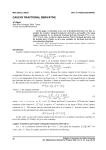Cauchy fractional derivative

Kaya U.

Статья научная

In this paper, we introduce a new sort of fractional derivative. For this, we consider the Cauchy's integral formula for derivatives and modify it by using Laplace transform. So, we obtain the fractional derivative formula F(α)(s) = L{(-1)(α)L-1{F(s)}}. Also, we find a relation between Weyl's fractional derivative and the formula above. Finally, we give some examples for fractional derivative of some elementary functions.

Бесплатно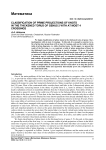Classification of prime projections of knots in the thickened torus of genus 2 with at most 4 crossings

Akimova A.A.

Статья научная

We begin classification of prime knots in the thickened torus of genus 2 having diagrams with at most 4 crossings. To this end, it is enough to construct a table of prime knot projections with at most 4 crossings, and use the table to obtain table of prime diagrams, i. e. table of prime knots. In this paper, we present the result of the first step, i. e. we construct a table of prime projections of knots in the thickened torus of genus 2 having at most 4 crossings. First, we introduce definition of prime projection of a knot in the thickened torus of genus 2. Second, we construct a table of prime projections of knots in the thickened torus of genus 2 having at most 4 crossings. To this end, we enumerate graphs of special type and consider all possible embeddings of the graphs into the torus of genus 2 that lead to prime projections. In order to simplify enumeration of the embeddings, we prove some auxiliary statements. Finally, we prove that all obtained projections are inequivalent. Several known and new tricks allow us to keep the process within reasonable limits and rigorously theoretically prove the completeness of the constructed table.

Бесплатно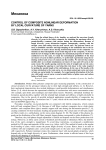Control of composite nonlinear deformation by local curvature of yarns

Sapozhnikov S.B., Kheruvimov A.V., Khoruzhiy A.S.

Статья научная

Using the refined theory of dry bundles, we analyzed the curvature (length diversity) of yarns in the fabric composite for obtaining the maximum effect of pseudo-ductility (constant yield stresses during the deformation). Because of length diversity, yarns destruction happens consequently, starting with the straight yarns and ending with the most curved ones. The polymer matrix ensures a monolithic structure and high damping of the oscillations due to the intensification of matrix shears during tension/compression of the composite. To simulate an ideal elastoplastic stress-strain diagram of the composite, we formulated the law of changes in fiber lengths, the mechanical properties of which were considered to be the same. To study the technological possibilities of the local curvatures of yarns in fabric preforms, we performed experiments using the indenting method and arrays of conical and flat needles. We showed that conical needles allow us to obtain simultaneous curvature of warp and weft yarns to the same extent. The needle spacing controls the ratio of straight and curved yarns; so, by changing the spacing we can bring this ratio closer to the value required for getting the needed length of the yield plateau. In the case of flat needles with various orientation to the warp yarns, we can obtain anisotropic yarns curvature (of only one yarns family, for example). For experimental deformation of the fabrics with locally curved yarns we used aramid fabrics of plain weave and carbon fabrics of twill weave.

Бесплатно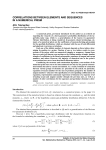Correlations between elements and sequences in a numerical prism

Tokmachev M.S.

Статья научная

A numerical prism, previously introduced by the author as an ordered set regarding the research of a three-parameter probability distribution of the hyperbolic-cosine type, which is a generalization of the known two-parameter Meixner distribution, is being considered. In geometry-related terminology, elements of a numerical prism are the coefficients of moment-forming polynomials for the specified distribution, which are obtained with the use of both differential and algebraic recurrence correlations. Each one of the infinite number of elements depends on three indices determining its position in a prism. Fixation of one or two indices results in cross-sections of the prism, which are numerical triangles or sequences. Among them, there are such well-known cross-sections as the Stirling number triangle, number triangle of coefficients in the Bessel polynomials, sequences of tangent and secant numbers, and others. However, the majority of numerical sets in the prism’s cross-sections have never been described in literature before. Considering the structure and construction algorithm, cross-sections of the numerical prism turn out to be interconnected not only by the general construction formula but also by certain correlations. As a result, formulas of connection between various groups of elements are presented in the article. In particular, expansion of secant numbers for the sum of products grouped by the number of tangent numbers’ cofactors with specification of corresponding coefficients in the expansion, representation (automatic expression) of elements of a sequence of alternating secant and tangent number through the previous ones, as well as a number of other correlations for the sequences and particular elements is determined.

Бесплатно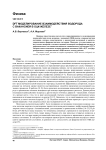DFT моделирование взаимодействия водорода с вакансией в ОЦК-железе

Верховых Анастасия Владимировна, Мирзоев Александр Аминулаевич

Статья научная

Впервые представлены результаты первопринципного моделирования взаимодействия атома водорода с вакансией ОЦК-железа в парамагнитном состоянии, которое сопоставляется с аналогичной величиной в магнитоупорядоченном ферромагнитном состоянии. Показано, что расстояния между атомом водорода и соответствующей октапорой для ферромагнитного и парамагнитного состояния оказываются одинаковыми и составляют 0,23 Å. Энергия связи комплекса водород-вакансия составила 0,60 и 0,27 для ферромагнитного и парамагнитного ОЦК-железа, соответственно.

Бесплатно

Журнал